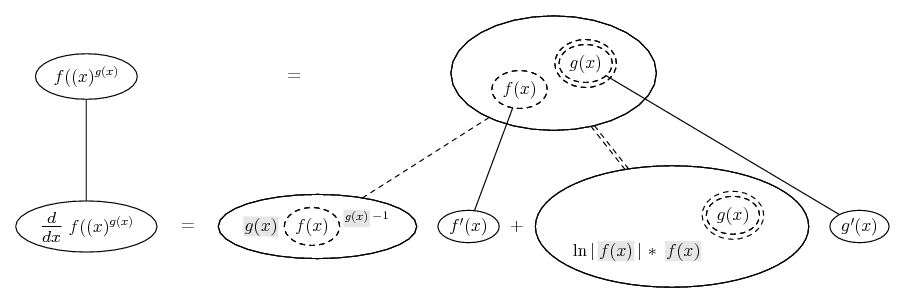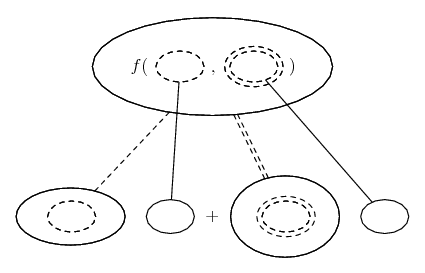# Thread: Derivative of f(x)^g(x)

1. ## Derivative of f(x)^g(x)

Here's a cute puzzle:

If you have the function $\displaystyle h(x)= f(x)^{g(x)}$, where f and g are differentiable functions, then there two mistakes one could make in trying to find the derivative of h.

1) Treat g(x) as if it were constant and use the "power rule" (very common): $\displaystyle (f^g)'= g(x)f(x)^{g(x)- 1}$.

2) tread f(x) as if it were constant and use the "exponential rule" (much less common): $\displaystyle (f^g)'= ln(f(x))(f(x)^{g(x)})g'(x)$.

Those are, as I said, wrong. Show that the correct derivative is the sum of those two mistakes!

2. OK, but we need an inner derivative in the first mistake, yes? Anyway, my take on it...... where... is the chain rule for two inner functions, i.e...

$\displaystyle \frac{d}{dx}\ f(u(x), v(x)) = \frac{\partial f}{\partial u} \frac{du}{dx} + \frac{\partial f}{\partial v} \frac{dv}{dx}$

As with...... the ordinary chain rule, straight continuous lines differentiate downwards (integrate up) with respect to x, and the straight dashed line similarly but with respect to the (corresponding) dashed balloon expression which is (one of) the inner function(s) of the composite expression.

Shading indicates an expression was treated as a constant on the way down (i.e. during partial differentiation).
_____________________________________

Don't integrate - balloontegrate!

Balloon Calculus Forum

Balloon Calculus Drawing with LaTeX and Asymptote!

3. All possible ambiguities are solved thanks to the identity...

$\displaystyle f(x)^{g(x)} = e^{g(x)\cdot \ln f(x)}$ (1)

From (1) we derive that...

$\displaystyle \frac{d}{dx} f(x)^{g(x)} = f(x)^{g(x)} \cdot \{\frac{g(x)\cdot f^{'}(x)}{f(x)} + g^{'}(x)\cdot \ln f(x)\}$ (2)

Kind regards

$\displaystyle \chi$ $\displaystyle \sigma$

#### Search Tags

derivative, fxgx